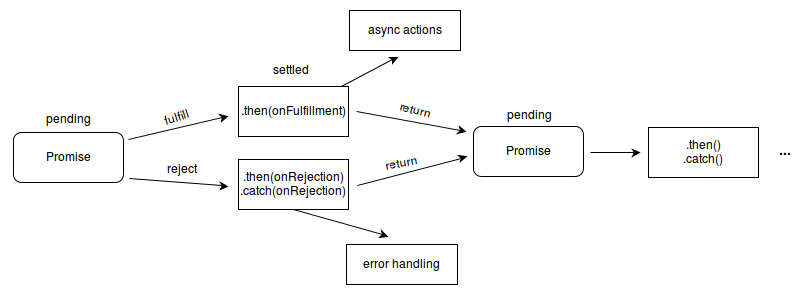X

# ES 6 / ES 2015 常用知识点归纳

#### 1. 迭代器和 for-of 循环：

``````function iteratableMethod() {
this.value = 0;
}

iteratableMethod.prototype[Symbol.iterator] = function() {
var self = this;

return {
next: function() {
var isDone = false;
if (self.value++ > 5)
isDone = true;
else
isDone = false;

return {
done: isDone,
value: self.value
}
}
}
``````

#### 2. let 和 const：

``````var attrs = [1, 2, 3];
for(let i = 0; i < attrs.length; i++) {
setTimeout(function() {
console.log(attrs[i]);
}, 2000 + 500 * i);
}
``````

#### 3. 不定参数和默认参数：

``````function method(name = "apple", price = 10, ...others) {
console.log(name);
console.log(price);
console.log(others);
}
``````

#### 4. 模板字符串：

``````let person = {
name: "Alice",
age: 18
};

let hometown = "USA";
// 标签模板；
let message = `I am \${person.name}, I'm from \${hometown}`;

console.log(message);
``````

#### 5. 箭头函数：

``````function method() {
return (a, b) => a + b;
}

function method() {
return (a, b) => {
return a + b;
}
}

function method() {
// 返回字面量对象时，要用小括号将对象包装起来；
return (a, b) => ({a, b});
}
``````

#### 6. Symbols：

Symbols 是 JS 的第七种原始类型，一般用于创建防止属性名冲突的属性，也就是说可以将 Symbols 作为对象的属性名，那么该属性名则不会与任一个属性名发生冲突。

``````var obj = {};
var mySymbol = Symbol("mySymbol");
obj[mySymbol] = 1;

Symbol.for("mySymbol") === Symbol.for("mySymbol") // true；
``````

#### 7. 解构：

``````let array = [1, 2, 3];
let [a, b, c] = array;

let {name: nameA} = {name: "Jason"};
let {missing} = {};

// 若没有变量声明符，则需要在整个表达式外部加小括号；
({blowUp} = {blowUp: 10});

// 设置默认值；
let [missing = true] = [];
let { message: msg = "Something went wrong" } = {};

// 导入 CommonJS 模块；
const { SourceMapConsumer, SourceNode } = require("source-map");
``````

#### 8. 生成器 Generator：

``````function* range(start, stop) {
for (var i = start; i < stop; i++)
yield i;
}

for(let item of range(1, 10)) {
console.log(item);
}

// 使任意对象可迭代；
function fai() {
this.i = [1, 2, 3, 4, 5];
}

fai.prototype.ite = function*() {
for(let item of this.i) {
yield item;
}
}

fai.prototype[Symbol.iterator] = function() {
return this.ite();
}
``````

#### 9. 类和子类：

ES6 中提供了类和子类的实现方法。

``````class Shape {
constructor(color) {
this._color = color;
};
}

class Circle extends Shape {
// 构造函数；
};
// 静态方法；
static draw(circle, canvas) {
// Canvas 绘制代码；
};
return !this._count ? 0 : this._count;
};
this._count = val;
};
// 实例方法；
area() {
};
};
throw new Error("圆的半径必须为整数。");
};
};
``````

#### 10. Promise：

Promise 主要用于解决“回调地狱”的问题，由于对于一个事务的各种情况都要在回调函数中进行处理，导致最终回调的方法一环套一环，失去了代码原有的可读性，并且这种回调的方式也不符合人们正常的逻辑思维方式。Promise/A+ 规范中规定 Promise 对象是一个有限状态机，它有三个状态，分别是从：pending -> fulfilledpending -> reject。Promise 的第一个事件节点的状态会沿着 Promise 链一直向下传递，如果其中某一个节点返回了 reject 状态的 Promise 对象或者抛出异常，则整个 Promise 的生命周期结束。我们可以通过在 Promise 链的最后加入 catch 方法来捕捉整个生命周期中发生的错误。`Promise.all`（Promise 全部 resolve 则响应） 和 `Promise.race`（响应最先 resolve 的 Promise）方法用来处理多并发事务的情况。``````var promise = new Promise((resolve, reject) => {
setTimeout(function() {
return resolve({data: "success1"});
}, 2000);
});

promise.then(data => {
console.log("success from promise 1: ", data);

return new Promise((resolve, reject) => {
setTimeout(function() {
return resolve({data: "success2"});
}, 2000);
});
}).then(data => {
console.log("success from promise 2: ", data);

return new Promise((resolve, reject) => {
setTimeout(function() {
return reject({data: "fail3"});
}, 2000);
});
}).then(data =>  {
console.log("success from promise 3: ", data);
}).catch(err => {
console.log("fail from promise 3: ", err);
});
``````

#### 11. 模块 Modules：

ES6 中的模块默认都是在严格模式下执的。

``````export function detectCats(canvas, options) {
var kittydar = new Kittydar(options);
return kittydar.detectCats(canvas);
}
export class Kittydar {
// ...
}
// 该函数没有被导出；
function resizeCanvas() {}
``````
``````import {detectCats, Kittydar} from "kittydar.js";
function go() {
var canvas = document.getElementById("catpix");
var cats = detectCats(canvas);
drawRectangles(canvas, cats);
}
``````